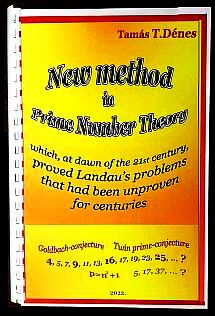Mathematics of T.Dénes Tamás   -------------------------------------- (01) Graph theoretical tools for the study of the cumulative build-up of empirical sociology (02) Sd-effect appearing in the genetic code, as the structural explanation of 20 triplets instead of 64 (03) Latin and magic squares from game applications to security (in Hungarian) (04) Latin squares and their applications in experimental design and coding (in Hungarian) (05) Latin squares and their applications in secrecy (in Hungarian) (06) Latin squares and codes (07) Complementary prime-sieve (C.P.S.) (pdf) (08) Complementary prime-sieve and a remark on S.W. Golomb’s factorization method (09) Estimation of the number of twin primes by application of Complementary Prime-sieve (10) Connections between the basic and the Fibonacci-type series (11) On the connections between RSA cryptosystem and the Fibonacci numbers (in Springer book) (12) Non associative algebraic system in cryptology (Protection against "meet in the middle" attack) (13) Cardan and cryptography (the mathematics of encryption grids) (14) GRAPH THEORETICAL APROACH TO STRUCTURAL REPRESENTATION OF SYSTEMS ----- (An attempt to generalize the holography principle) Kyoto, Japan, 1978. (15) STRUCTURE MEMORY (SM) as a universal graph model of the human memory (16) Dénes type Symmetric Prime Number theorem and its application to proof of the Even Goldbach conjecture (17) Application of the Dénes type Symmetric Prime Number theorem to proof of there exist infinitely many primes of the form n2+1 (18) Proof of the Twin prime conjecture (19) Generalized Twin prime theorem (in Hungarian) (20) Proof of the existence of prime number between successive squares (in Hungarian) (21) The non-publishability theorem of the proof of Goldbach's conjecture or ----- A story about the possibility of mathematics not being an exact science after all (22) Basic properties of Mersenne-numbers (Parallel algorithm for prime factorization of Mersenne-numbers) (23) Properties of twin prime-based Mersenne twin primes (24) Proof of the existence of infinite number of Mersenne primesNew method in Prime Number Theory which, at dawn of the 21st century, proved Landau's problems that had been unproven for centuries